xFixed point (mathematics)Encyclopedia
In mathematics
Mathematics
Mathematics is the study of quantity, space, structure, and change. Mathematicians seek out patterns and formulate new conjectures. Mathematicians resolve the truth or falsity of conjectures by mathematical proofs, which are arguments sufficient to convince other mathematicians of their validity...

, a fixed point (sometimes shortened to fixpoint, also known as an invariant point) of a function
Function (mathematics)
In mathematics, a function associates one quantity, the argument of the function, also known as the input, with another quantity, the value of the function, also known as the output. A function assigns exactly one output to each input. The argument and the value may be real numbers, but they can...

is a point that is mapped to itself by the function. A set of fixed points is sometimes called a fixed set. That is to say, c is a fixed point of the function f(x) if and only if
If and only if
In logic and related fields such as mathematics and philosophy, if and only if is a biconditional logical connective between statements....

f(c) = c.
For example, if f is defined on the real number
Real number
In mathematics, a real number is a value that represents a quantity along a continuum, such as -5 , 4/3 , 8.6 , √2 and π...

s by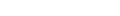then 2 is a fixed point of f, because f(2) = 2.

Not all functions have fixed points: for example, if f is a function defined on the real numbers as f(x) = x + 1, then it has no fixed points, since x is never equal to x + 1 for any real number. In graphical terms, a fixed point means the point (x, f(x)) is on the line y = x, or in other words the graph
Graph of a function
In mathematics, the graph of a function f is the collection of all ordered pairs . In particular, if x is a real number, graph means the graphical representation of this collection, in the form of a curve on a Cartesian plane, together with Cartesian axes, etc. Graphing on a Cartesian plane is...

of f has a point in common with that line. The example f(x) = x + 1 is a case where the graph and the line are a pair of parallel
Parallel (geometry)
Parallelism is a term in geometry and in everyday life that refers to a property in Euclidean space of two or more lines or planes, or a combination of these. The assumed existence and properties of parallel lines are the basis of Euclid's parallel postulate. Two lines in a plane that do not...

lines.

Points which come back to the same value after a finite number of iterations
Iterated function
In mathematics, an iterated function is a function which is composed with itself, possibly ad infinitum, in a process called iteration. In this process, starting from some initial value, the result of applying a given function is fed again in the function as input, and this process is repeated...

of the function are known as periodic point
Periodic point
In mathematics, in the study of iterated functions and dynamical systems, a periodic point of a function is a point which the system returns to after a certain number of function iterations or a certain amount of time.- Iterated functions :...

s; a fixed point is a periodic point with period equal to one.

## Attractive Fixed Points

An attractive fixed point of a function f is a fixed point x0 of f such that for any value of x in the domain that is close enough to x0, the iterated function
Iterated function
In mathematics, an iterated function is a function which is composed with itself, possibly ad infinitum, in a process called iteration. In this process, starting from some initial value, the result of applying a given function is fed again in the function as input, and this process is repeated...

sequence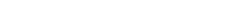converges
Limit of a sequence
The limit of a sequence is, intuitively, the unique number or point L such that the terms of the sequence become arbitrarily close to L for "large" values of n...

to x0. An expression of prerequisites and proof of the existence of such solution is given by Banach fixed point theorem
Banach fixed point theorem
In mathematics, the Banach fixed-point theorem is an important tool in the theory of metric spaces; it guarantees the existence and uniqueness of fixed points of certain self-maps of metric spaces, and provides a constructive method to find those fixed points...

.

The natural cosine function ("natural" means in radians, not degrees or other units) has exactly one fixed point, which is attractive. In this case, "close enough" is not a stringent criterion at all—to demonstrate this, start with any real number and repeatedly press the cos key on a calculator (checking first that the calculator is in "radians" mode). It eventually converges to about 0.739085133, which is a fixed point. That is where the graph of the cosine function intersects the line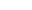.

Not all fixed points are attractive: for example, x = 0 is a fixed point of the function f(x) = 2x, but iteration of this function for any value other than zero rapidly diverges. However, if the function f is continuously differentiable in an open neighbourhood of a fixed point x0, and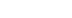, attraction is guaranteed.

Attractive fixed points are a special case of a wider mathematical concept of attractor
Attractor
An attractor is a set towards which a dynamical system evolves over time. That is, points that get close enough to the attractor remain close even if slightly disturbed...

s.

An attractive fixed point is said to be a stable fixed point if it is also Lyapunov stable.

A fixed point is said to be a neutrally stable fixed point if it is Lyapunov stable but not attracting. The center of a linear homogeneous differential equation of the second order is an example of a neutrally stable fixed point.

## Theorems guaranteeing fixed points

There are numerous theorems in different parts of mathematics that guarantee that functions, if they satisfy certain conditions, have at least one fixed point. These are amongst the most basic qualitative results available: such fixed-point theorem
Fixed-point theorem
In mathematics, a fixed-point theorem is a result saying that a function F will have at least one fixed point , under some conditions on F that can be stated in general terms...

s that apply in generality provide valuable insights.

## Applications

In many fields, equilibria or stability
Stability theory
In mathematics, stability theory addresses the stability of solutions of differential equations and of trajectories of dynamical systems under small perturbations of initial conditions...

are fundamental concepts that can be described in terms of fixed points. For example, in economics
Economics
Economics is the social science that analyzes the production, distribution, and consumption of goods and services. The term economics comes from the Ancient Greek from + , hence "rules of the house"...

, a Nash equilibrium
Nash equilibrium
In game theory, Nash equilibrium is a solution concept of a game involving two or more players, in which each player is assumed to know the equilibrium strategies of the other players, and no player has anything to gain by changing only his own strategy unilaterally...

of a game
Game theory
Game theory is a mathematical method for analyzing calculated circumstances, such as in games, where a person’s success is based upon the choices of others...

is a fixed point of the game's best response correspondence
Best response
In game theory, the best response is the strategy which produces the most favorable outcome for a player, taking other players' strategies as given...

. However, in physics, more precisely in the theory of Phase Transitions
Phase transition
A phase transition is the transformation of a thermodynamic system from one phase or state of matter to another.A phase of a thermodynamic system and the states of matter have uniform physical properties....

, linearisation near an unstable fixed point has led to Wilson
Kenneth G. Wilson
Kenneth Geddes Wilson is an American theoretical physicist and Nobel Prize winner.As an undergraduate at Harvard, he was a Putnam Fellow. He earned his PhD from Caltech in 1961, studying under Murray Gell-Mann....

's Nobel prize-winning work inventing the renormalization group
Renormalization group
In theoretical physics, the renormalization group refers to a mathematical apparatus that allows systematic investigation of the changes of a physical system as viewed at different distance scales...

, and to the mathematical explanation of the term "critical phenomenon".

In compilers, fixed point computations are used for whole program analysis, which are often required to do code optimization
Optimization (computer science)
In computer science, program optimization or software optimization is the process of modifying a software system to make some aspect of it work more efficiently or use fewer resources...

.

The vector of PageRank
PageRank
PageRank is a link analysis algorithm, named after Larry Page and used by the Google Internet search engine, that assigns a numerical weighting to each element of a hyperlinked set of documents, such as the World Wide Web, with the purpose of "measuring" its relative importance within the set...

values of all web pages is the fixed point of a linear transformation
Linear transformation
In mathematics, a linear map, linear mapping, linear transformation, or linear operator is a function between two vector spaces that preserves the operations of vector addition and scalar multiplication. As a result, it always maps straight lines to straight lines or 0...

derived from the World Wide Web
World Wide Web
The World Wide Web is a system of interlinked hypertext documents accessed via the Internet...

Logician Saul Kripke
Saul Kripke
Saul Aaron Kripke is an American philosopher and logician. He is a professor emeritus at Princeton and teaches as a Distinguished Professor of Philosophy at the CUNY Graduate Center...

makes use of fixed points in his influential theory of truth. He shows how one can generate a partially defined truth predicate (one which remains undefined for problematic sentences like "This sentence is not true"), by recursively defining "truth" starting from the segment of a language which contains no occurrences of the word, and continuing until the process ceases to yield any newly well-defined sentences. (This will take a denumerable infinity of steps.) That is, for a language L, let L-prime be the language generated by adding to L, for each sentence S in L, the sentence "S is true." A fixed point is reached when L-prime is L; at this point sentences like "This sentence is not true" remain undefined, so, according to Kripke, the theory is suitable for a natural language which contains its own truth predicate.

The concept of fixed point can be used to define the convergence
Limit of a function
In mathematics, the limit of a function is a fundamental concept in calculus and analysis concerning the behavior of that function near a particular input....

of a function.

## Topological fixed point property

A topological space
Topological space
Topological spaces are mathematical structures that allow the formal definition of concepts such as convergence, connectedness, and continuity. They appear in virtually every branch of modern mathematics and are a central unifying notion...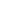is said to have the fixed point property (briefly FPP) if for any continuous function
Continuous function
In mathematics, a continuous function is a function for which, intuitively, "small" changes in the input result in "small" changes in the output. Otherwise, a function is said to be "discontinuous". A continuous function with a continuous inverse function is called "bicontinuous".Continuity of...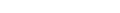there exists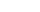such that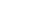.

The FPP is a topological invariant, i.e. is preserved by any homeomorphism
Homeomorphism
In the mathematical field of topology, a homeomorphism or topological isomorphism or bicontinuous function is a continuous function between topological spaces that has a continuous inverse function. Homeomorphisms are the isomorphisms in the category of topological spaces—that is, they are...

. The FPP is also preserved by any retraction.

According to the Brouwer fixed point theorem
Brouwer fixed point theorem
Brouwer's fixed-point theorem is a fixed-point theorem in topology, named after Luitzen Brouwer. It states that for any continuous function f with certain properties there is a point x0 such that f = x0. The simplest form of Brouwer's theorem is for continuous functions f from a disk D to...

, every compact
Compact space
In mathematics, specifically general topology and metric topology, a compact space is an abstract mathematical space whose topology has the compactness property, which has many important implications not valid in general spaces...

and convex
Convex set
In Euclidean space, an object is convex if for every pair of points within the object, every point on the straight line segment that joins them is also within the object...

subset of a euclidean space
Euclidean space
In mathematics, Euclidean space is the Euclidean plane and three-dimensional space of Euclidean geometry, as well as the generalizations of these notions to higher dimensions...

has the FPP. Compactness alone does not imply the FPP and convexity is not even a topological property so it makes sense to ask how to topologically characterize the FPP. In 1932 Borsuk
Karol Borsuk
Karol Borsuk was a Polish mathematician.His main interest was topology.Borsuk introduced the theory of absolute retracts and absolute neighborhood retracts , and the cohomotopy groups, later called Borsuk-Spanier cohomotopy groups. He also founded the so called Shape theory...

asked whether compactness together with contractibility
Contractible space
In mathematics, a topological space X is contractible if the identity map on X is null-homotopic, i.e. if it is homotopic to some constant map. Intuitively, a contractible space is one that can be continuously shrunk to a point....

could be a necessary and sufficient condition for the FPP to hold. The problem was open for 20 years until the conjecture was disproved by Kinoshita who found an example of a compact contractible space without the FPP.